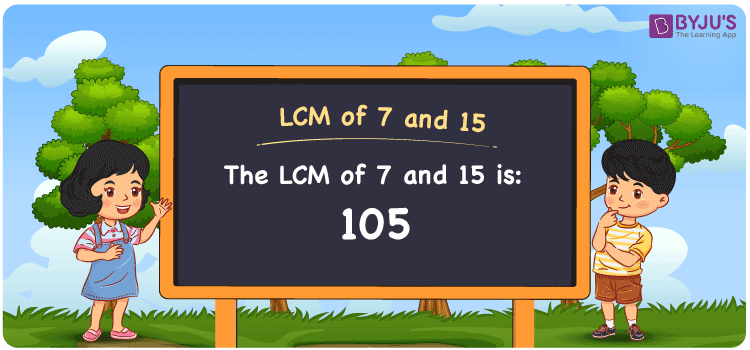Checkout JEE MAINS 2022 Question Paper Analysis : Checkout JEE MAINS 2022 Question Paper Analysis :

# LCM of 7 and 15

LCM of 7 and 15 is 105. Using the multiples of 7 and 15, the value which is evenly divisible by 7 and 15 gives the LCM value. Least common multiple of 7 and 15 is the common multiple we obtain with the help of the multiplication operation. (7, 14, 21, 28, 35, ….) and (15, 30, 45, 60, 75, ….) are the multiples of 7 and 15. Students can learn how the LCM of two numbers is found with the help of listing multiples, prime factorization and division.

## What is LCM of 7 and 15?

The answer to this question is 105. The LCM of 7 and 15 using various methods is shown in this article for your reference. The LCM of two non-zero integers, 7 and 15, is the smallest positive integer 105 which is divisible by both 7 and 15 with no remainder.## How to Find LCM of 7and 15?

LCM of 7 and 15 can be found using three methods:

• Prime Factorisation
• Division method
• Listing the multiples

### LCM of 7 and 15 Using Prime Factorisation Method

The prime factorisation of 7 and 15, respectively, is given by:

7 = 7¹

15 = 3 x 5 = 3¹ x 5¹

LCM (7, 15) = 105

### LCM of 7 and 15 Using Division Method

We’ll divide the numbers (7, 15) by their prime factors to get the LCM of 7 and 15 using the division method (preferably common). The LCM of 7 and 15 is calculated by multiplying these divisors.

 3 7 15 5 7 5 7 7 1 x 1 1

No further division can be done.

Hence, LCM (7, 15) = 105

### LCM of 7 and 15 Using Listing the Multiples

To calculate the LCM of 7 and 15 by listing out the common multiples, list the multiples as shown below

 Multiples of 7 Multiples of 15 7 15 14 30 21 45 28 60 35 75 42 90 49 105 56 120 63 135 70 150 77 165 84 180 91 195 98 205 105 220

LCM (7, 15) = 105

## Video Lesson on Applications of LCM## LCM of 7 and 15 Solved Examples

What is the smallest number exactly divisible by 7 and 15?

Solution:

We know that

LCM is the smallest number exactly divisible by 7 and 15.

Multiples of 7 = 7, 14, 21, 28, 35, 42, ….

Multiples of 15 = 15, 30, 45, 60, 75, 90, …..

Hence, the LCM of 7 and 15 is 105.

## Frequently Asked Questions on LCM of 7 and 15

### Show the methods used to determine the LCM of 7 and 15.

The methods used to determine the LCM of 7 and 15 are

Prime Factorisation

Division method

Listing the multiples

### With the help of the prime factorisation method, find the LCM of 7 and 15.

First we have to analyze the factors in order to determine the LCM

7 = 7¹

15 = 3 x 5 = 3¹ x 5¹

LCM is the product of prime factors raised to the highest exponent among 7 and 15.

LCM of 7 and 15 = 105

### Find the GCF if the LCM of 7 and 15 is 105.

LCM x GCF = 7 x 15

As the LCM = 105

105 x GCF = 105

GCF = 105/105 = 1